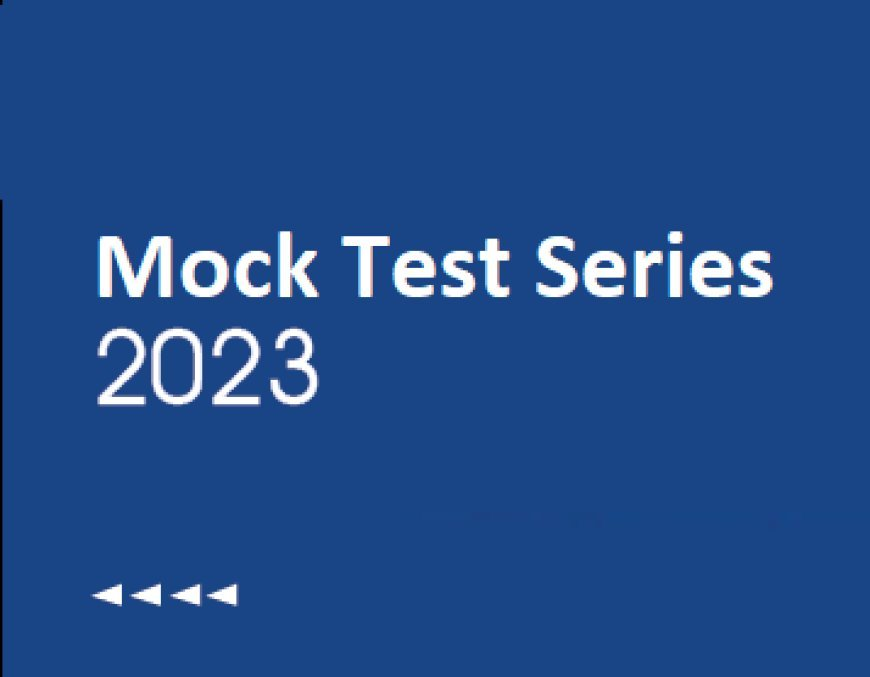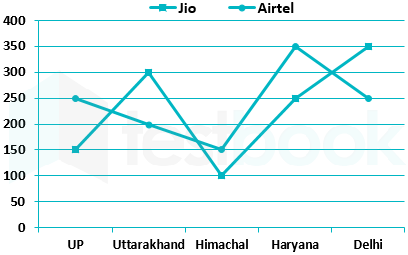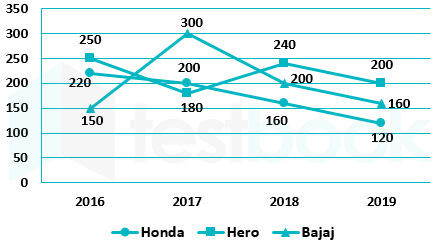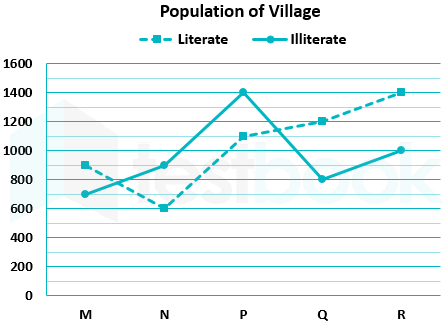# AAO Exam-CT 10: Quant (DI - Line Graph)

## AAO Exam-CT 10- Quant (DI - Line Graph)

0  6191. Directions: Study the chart and answer the following questions.

The line graph show number of Jio and Airtel users in 5 different states.Question:

How many total Airtel users are there in all five states together?

• 1250
• 1200
• 1150
• 1000
• None of these

Given:

Number of Airtel users in UP = 250

Number of Airtel users in Uttarakhand = 200

Number of Airtel users in Himachal = 150

Number of Airtel users in Haryana = 350

Number of Airtel users in Delhi = 250

Calculations:

Total Airtel users in all five states together = 250 + 200 + 150 + 350 + 250

⇒ 1200

∴ Total Airtel users in all five states together are 1200

2. Directions: Study the chart and answer the following questions.

The line graph show number of Jio and Airtel users in 5 different states.Question:
What is the average of Jio users in Delhi, Uttarakhand and Haryana?
• 900
• 300
• 600
• 200
• None of these

Given:

Number of Jio users in Delhi = 350

Number of Jio users in Uttarakhand = 300

Number of Jio users in Haryana = 250

Formula used:

Average = Sum of values/Number of values

Calculations:

Average of Jio users in Delhi, Uttarakhand and Haryana = (350 + 300 + 250)/3

⇒ 900/3

⇒ 300

∴ The average of Jio users in Delhi, Uttarakhand and Haryana is 300

3. Directions: Study the chart and answer the following questions.

The line graph show number of Jio and Airtel users in 5 different states.Question:

What is the ratio of Jio users in Delhi to Airtel users in UP?

• 8 : 5
• 5 : 8
• 7 : 5
• 5 : 7
• None of these

Given:

Number of Jio users in Delhi = 350

Number of Airtel users in UP = 250

Calculations:

Ratio of Jio users in Delhi to Airtel users in UP = 350 : 250

⇒ 7 : 5

∴ The ratio of Jio users in Delhi to Airtel users in UP is 7 : 5

4. Direction: Following line graph shows the number of bikes sold by different companies in different years.Question:
If price of Honda bike is 20% more than that of hero bike so what is ratio of revenue generated by Honda to hero in year 2017?
• 3 : 8
• 5 : 7
• 6 : 1
• 5 : 3
• 4 : 3

Let the price of hero bike be 100a so, price of Honda bike is 120a

Revenue generated by hero bike in 2017 = 100a × 180

Revenue generated by Honda bike in 2017 = 120a × 200

∴ according to question

120a × 200 : 100a × 180

⇒ required ratio 4 : 3

5. Direction: Following line graph shows the number of bikes sold by different companies in different years.Question:
if bikes sold in 2018 is 20% less than the bikes sold in 2020. Find the number of bikes sold in 2020?
• 650
• 750
• 800
• 900
• 850

Bikes sold in 2018 = 160 + 240 + 200 = 600

⇒ Required solution = 600/(100 – 20) × 100

600/80 × 100 = 750

6. Direction: Following line graph shows the number of bikes sold by different companies in different years.Question:
What is the ratio of bikes sold in the year 2016 to the bikes sold in the year 2019?
• 24 : 31
• 23 : 30
• 31 : 11
• 31 : 24
• none of these

Bikes sold in year 2016 = 220 + 250 + 150 = 620

Bikes sold in year 2019 = 120 + 200 + 160 = 480

∴ Required ratio = 31 : 24

7. Direction: Following line graph shows the number of bikes sold by different companies in different years.Question:
Bikes sold by hero and Bajaj together in the year 2016 is how much percent more/less than the number of Bajaj bikes sold in 2017.
• 30%
• 45%
• 33.33%
• 50%
• 20%

Bikes sold by hero in 2016 = 250

Bikes sold by Bajaj in 2016 = 150

Sum of bikes sold by hero and Bajaj in 2016 = 250 + 150 = 400

Bikes sold by Bajaj in 2017 = 300

∴ Required percentage = (400 – 300)/300 × 100 = 1/3 × 100 = 33.33%

8. Directions: Study the following data and answer the following questions:

Following line graph shows the literate and illiterate population of five villages.

Total population = literate population + illiterate populationQuestion:
Find the ratio between the total population of village N and village R.
• 1 : 3
• 6 : 7
• 9 : 1
• 5 : 8
• 8 : 9

Given:

Total population of village N = 600 + 900 = 1500

Total population of village R = 1400 + 1000 = 2400

Required ratio =

= 1500 : 2400

= 5 : 8

9. Directions: Study the following data and answer the following questions:

Following line graph shows the literate and illiterate population of five villages.

Total population = literate population + illiterate populationQuestion:
The literate population of village Q is approximately what percent of the total population of village Q?
• 75%
• 55%
• 65%
• 60%
• 70%

Given:

Total population of village Q = 1200 + 800 = 2000

Total literate population of village Q = 1200

Required percentage =

= 1200/2000 × 100

= 60%

10. Directions: Study the following data and answer the following questions:

Following line graph shows the literate and illiterate population of five villages.

Total population = literate population + illiterate populationQuestion:
Find the average Literate population of five villages.
• 1100
• 1200
• 1250
• 1360
• 1040

Given:

Total literate population of five villages =

= 900 + 600 + 1100 + 1200 + 1400 = 5200

Required average = 5200/5 = 1040

### AAO Exam-CT 11: Quant (Average)